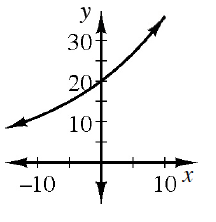### Home > INT2 > Chapter 12 > Lesson 12.2.2 > Problem12-89

12-89.

For the exponential function $y = 20(1.06)^x$:

1. What are the starting point ($y$-intercept) and multiplier? Sketch a graph.2. What situation could this function describe?

The initial value is the $y$-intercept.